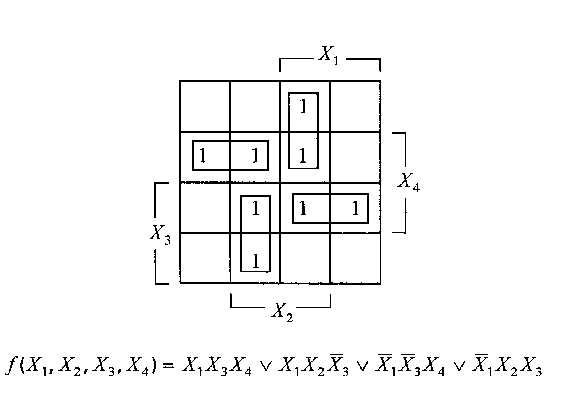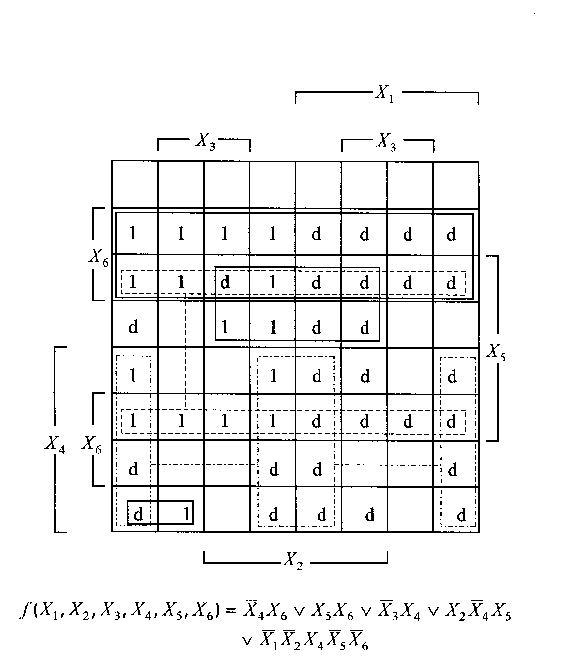# Karnaugh map

A graphic representation of sets, formulas of mathematical logic, events of probability theory, and statements or propositions concerning Boolean algebras or any isomorphic entities thereof (cf. also Boolean algebra). A classical Karnaugh map of $n$ variables is a two-dimensional display of a Boolean or switching function $f : {B ^ {n} } \rightarrow B$, where $B$ is the two-element Boolean algebra $\{ 0,1 \}$ or $\{ \textrm{ false } , \textrm{ true } \}$. The map is usually drawn as a rectilinear figure of $2 ^ {n}$ cells whose boundaries are rectilinear segments, so that a cell will have a square (or, sometimes, triangular) contour. The combinations of the input map variables (usually called discriminants of the map function) are ordered according to the reflected binary code (known also as the Gray code). This ordering gives the map the visual advantage that neighbouring cells are represented by adjacent input variable combinations, i.e., by binary numbers that differ in only one bit position. The top and bottom rows of the map are viewed as contiguous. Similarly, the leftmost and rightmost columns are considered adjacent. In that sense, a Karnaugh map can be imagined to exist on the surface of a torus, not that of a plane.

The Karnaugh map is particularly useful in the representation and manipulation of incompletely-specified switching functions (also called partial functions), of the form $f : X \rightarrow B$, where $X \subset B ^ {n}$. When a Karnaugh map is drawn, it is usually implicitly assumed that its $n$ input variables are independent. However, the map can be readily modified to handle certain variable dependencies (such as certain cell mutual exclusions), which results in reducing the number of cells to less than $2 ^ {n}$.

The Karnaugh map is most useful for functions of $4$ or fewer variables, because the cells for which a given variable is assigned the value $1$ form a contiguous band. For maps of more than $4$ variables, not all variables are associated with such bands, but as much visual help as possible is offered. Therefore, the map is used normally to handle up to $6$ variables only, though it may be used, with increasing complexity, to handle up to $9$ variables. With slight modifications, however, the map can be conveniently used to handle more variables.

There are several entities that the Karnaugh map resembles. The Karnaugh map is simply a different way of representing a truth table. However, it is more concise, saves time, space and effort and gives a pictorial insight to many algebraic concepts. The Venn diagram (Euler diagram) offers the visual help of the Karnaugh map. However, it is essentially a curvilinear figure whose boundaries are closed and without self-intersections. The widespread practice is to draw these boundaries as convex curves such as circles or ellipses. This unfortunate practice unnecessarily limits the use of the Venn diagram to $4$ variables only. Both the Carroll diagram and the Karnaugh map are rectilinear figures that offer good visual aid by keeping subdivisions of any general class within one boundary. In fact, they are exactly the same for $n = 2$. Carroll diagrams can handle up to $10$ variables, but they seem to have not been much explored. The ancestor of the Karnaugh map is the logical diagram proposed by A. Marquand and rediscovered by E.W. Veitch as a logical chart. The Marquand–Veitch diagram lacks the property of adjacency of rows, columns and cells enjoyed by the Karnaugh map.

There are map types other than the classical or conventional type. The variable-entered Karnaugh map has been developed to double the variable-handling capability of the classical map. Its input consists of $m$ variables, called map variables, while its entries are Boolean formulas or functions of $n - m$ variables, called entered variables. Hence, it can be used to represent "big" Boolean functions of the form $f : {B ^ {n - m } } \rightarrow B$, where $B = \{ 0,1 \}$, or of the form $f : {B ^ {k} } \rightarrow B$, where $B = \{ 0,1 \} ^ {j}$, $j > 1$, is the Boolean algebra of $2 ^ {j}$ elements. A Karnaugh map of real entries is used to represent pseudo-switching (pseudo-Boolean) functions of the form $f : {B ^ {n} } \rightarrow \mathbf R$, where $\mathbf R$ is the field of real numbers. The Karnaugh map is also useful as a probability map for representing probability functions $f : {X ^ {n} } \rightarrow X$, where $X = [ 0.0,1.0 ] \subset \mathbf R$.

The Karnaugh map is widely used by logical designers. It is discussed in almost every text on logic design and switching theory. It can serve as a pictorial and pedagogical demonstration of basic switching theory concepts such as duality, prime implicants and essential prime implicants. In addition, it can be used for implementing Boolean operations collectively on significant portions of the input domain without going down to the cell level. The most famous use of the map is for minimization of a switching function, i.e., to obtain a formula for the function in sum-of-products (disjunction of conjunctions) form that has a minimum number of prime implicants as the primary objective and that has a minimum number of literals as the secondary objective. The map is also used for obtaining the minimal dual form, i.e., the minimal product-of-sums (conjunction of disjunctions) form. The map finds other uses in the complementation, differencing (the so-called "differentiation" ) and spectral-coefficient evaluation of switching functions, symbolic reliability analysis, solution of Boolean equations, and the like.Figure: k110040a

A Karnaugh map for a $4$- variable completely specified switching function.Figure: k110040b

A Karnaugh map for a $6$- variable incompletely specified switching function. The $d$ entries stand for don't cares, and the empty cells are understood to contain $0$.

How to Cite This Entry:
Karnaugh map. Encyclopedia of Mathematics. URL: http://encyclopediaofmath.org/index.php?title=Karnaugh_map&oldid=47479
This article was adapted from an original article by A.M. Rushdi (originator), which appeared in Encyclopedia of Mathematics - ISBN 1402006098. See original article